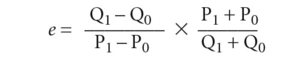# arc elasticity

Also found in: Wikipedia.

## Arc Elasticity

A variable's elasticity (or relative stability of a variable with respect to another variable's change) when the two variables are between two given points. One calculates the arc elasticity by dividing the percentage change of one variable by the percentage change of the other. This is used to find a more accurate measure of the price elasticity of demand.

## arc elasticity

a rough measure of the responsiveness of DEMAND or SUPPLY to changes in PRICE, INCOME, etc. In the case of PRICE ELASTICITY OF DEMAND, it is the ratio of the percentage change in quantity demanded (Q) to the percentage change in price (P) over a price range such as P0 to P1 in Fig. 8. Arc elasticity of demand is expressed notationally as:where P0 = original price, Q0 = original quantity, P1 = new price, Q1 = new quantity. Because arc elasticity measures the elasticity of demand (e) over a price range or arc of the demand curve, it is only an approximation of demand elasticity at a particular price (POINT ELASTICITY). However, the arc elasticity formula gives a reasonable degree of accuracy in approximating point elasticity when price and/or quantity changes are small. See also ELASTICITY OF DEMAND.

Collins Dictionary of Economics, 4th ed. © C. Pass, B. Lowes, L. Davies 2005
Mentioned in ?
References in periodicals archive ?
The results of the sensitivity analysis show that the arc elasticity of the vacant distance is 0.034, whereas the arc elasticity of the occupied distance is 0.028.
In particular, we compute arc elasticity around the equilibrium market price for each hour.
As outlined by Vazquez (2008), discussion and debate on the accuracy and applicability of Allen's arc elasticity has a long history, dating back to the 1930s and continuing into the 1980s, 1990s, and 2000s (e.g., Vazquez 1985; Daellenbach et al.
Price elasticity of demand was then estimated by dividing the estimated percent change in number of prescriptions, based on the cap effect estimated in (1), by the estimated percentage change in beneficiaries' out-of-pocket price for prescription drugs, basing the percentage at the midpoint of the pre- and postcap quantifies and prices (arc elasticity).
One way of summarizing price responsiveness when price changes are large is the arc elasticity. Arc elasticity equals the percentage change in energy use relative to the average of the new and old values for both quantity (Q) and price (P), as depicted in the following equation:
An arc elasticity method was then used to account for the endpoints of each income range.
Unfortunately, the concept of arc elasticity does not allow for an unambiguous ranking of the two markets: For small price intervals around the kink in the demand curves the arc elasticity ranks the elasticity of the first market lower, but for larger intervals and for intervals that do not contain that price, in particular, for intervals around either of the critical prices at which the firm may consider pricing, the ranking is in line with the results from the use of the more common point elasticity.
Since "arc elasticity" represents a popular measurement of economists, it also would have been interesting to know how marketers can determine inflection points, and where limits of marketing influence are reached.
Solving Equation 12 for [[Gamma].sub.1] and recognizing that the arc elasticity [e.sub.S] is [R.sub.i]/[R.sub.G] shows that if [[Gamma].sub.0] = 0 then [[Gamma].sub.1] is [e.sub.S].
In their recent article in this journal, "Restrictions of Allen's Arc Elasticity of Demand; Time to Consider the Alternative?" Daellenbach, Khandker, Knowles, and Sherony|l991~ (hereafter "DKKS") join a long list of detractors of Allen's |1934~ arc elasticity formula.
The critical arc elasticity of demand between the initial price and the monopoly prices is given by 1(r + t/50).

Site: Follow: Share:
Open / Close